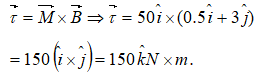# NEET Questions Solved

A magnet of magnetic moment  is placed along the x-axis in a magnetic field $\stackrel{\to }{B}=\left(0.5\stackrel{^}{i}+3.0\stackrel{^}{j}\right)T$. The torque acting on the magnet is

(a) 175 $\stackrel{^}{k}$ N-m

(b) 150 $\stackrel{^}{k}$ N-m

(c) 75 $\stackrel{^}{k}$ N-m

(d) 25$\sqrt{37}$ $\stackrel{^}{k}$ N-mDifficulty Level:

• 15%
• 54%
• 19%
• 15%